# Introduction

We will take a dive into the ‘College’ data set from the ISLR library in R. The data set contains statistics for a large number of US Colleges from the 1995 issue of US News and World Report. There are 18 variables and 777 observations. This dataset was taken from the StatLib library which is maintained at Carnegie Mellon University. The dataset was used in the ASA Statistical Graphics Section’s 1995 Data Analysis Exposition.

### Attributes:

[Private] Whether the college is public or private
[Accept] Number of applications accepted
[Enroll] Number of new students enrolled
[Top10perc] Percentage of new students from top 10% of High School class
[Top25perc] Percentage new students from top 25% of High School class
[Outstate] Out-of-state tuition
[Room.Board] Room and board costs
[Books] Estimated book costs
[Personal] Estimated personal spending
[PhD] Percentage of faculty with Ph.D.’s
[Terminal] Percentage of faculty with terminal degree

This data is used to compare colleges in the United States. In most respects, the colleges are fairly varied and provide a range of opportunities to suit a similarly varied student population. The cost of college and the expenditure by the college on the student, however, is skewed. These are variables that may be worth further analysis, given concern over the rising cost of college and university. The colleges with strong outcomes for students also typically have a high cost. This analysis will use the total instructional expenditure as a proportion of the total cost of attending the college as a measure of cost effectiveness, taking into account qualititative variables such as student to faculty ratio and graduation rate.

First, let us load the ISLR library, so we can access the ‘College’ data set.

``library(ISLR)``

We first take a look at the structure of our data by using str() function in R.

``str(College)``
``````## 'data.frame':    777 obs. of  18 variables:
##  \$ Private    : Factor w/ 2 levels "No","Yes": 2 2 2 2 2 2 2 2 2 2 ...
##  \$ Apps       : num  1660 2186 1428 417 193 ...
##  \$ Accept     : num  1232 1924 1097 349 146 ...
##  \$ Enroll     : num  721 512 336 137 55 158 103 489 227 172 ...
##  \$ Top10perc  : num  23 16 22 60 16 38 17 37 30 21 ...
##  \$ Top25perc  : num  52 29 50 89 44 62 45 68 63 44 ...
##  \$ F.Undergrad: num  2885 2683 1036 510 249 ...
##  \$ P.Undergrad: num  537 1227 99 63 869 ...
##  \$ Outstate   : num  7440 12280 11250 12960 7560 ...
##  \$ Room.Board : num  3300 6450 3750 5450 4120 ...
##  \$ Books      : num  450 750 400 450 800 500 500 450 300 660 ...
##  \$ Personal   : num  2200 1500 1165 875 1500 ...
##  \$ PhD        : num  70 29 53 92 76 67 90 89 79 40 ...
##  \$ Terminal   : num  78 30 66 97 72 73 93 100 84 41 ...
##  \$ S.F.Ratio  : num  18.1 12.2 12.9 7.7 11.9 9.4 11.5 13.7 11.3 11.5 ...
##  \$ perc.alumni: num  12 16 30 37 2 11 26 37 23 15 ...
##  \$ Expend     : num  7041 10527 8735 19016 10922 ...
##  \$ Grad.Rate  : num  60 56 54 59 15 55 63 73 80 52 ...``````

As we can see, there are 17 quantitative variables and 1 qualitative variable. Then we use summary() function for quantitative variables and table() function for the qualitative variable to generate a numeric summary of the variables.

``summary(College)``
``````##  Private        Apps           Accept          Enroll       Top10perc
##  No :212   Min.   :   81   Min.   :   72   Min.   :  35   Min.   : 1.00
##  Yes:565   1st Qu.:  776   1st Qu.:  604   1st Qu.: 242   1st Qu.:15.00
##            Median : 1558   Median : 1110   Median : 434   Median :23.00
##            Mean   : 3002   Mean   : 2019   Mean   : 780   Mean   :27.56
##            3rd Qu.: 3624   3rd Qu.: 2424   3rd Qu.: 902   3rd Qu.:35.00
##            Max.   :48094   Max.   :26330   Max.   :6392   Max.   :96.00
##  Min.   :  9.0   Min.   :  139   Min.   :    1.0   Min.   : 2340
##  1st Qu.: 41.0   1st Qu.:  992   1st Qu.:   95.0   1st Qu.: 7320
##  Median : 54.0   Median : 1707   Median :  353.0   Median : 9990
##  Mean   : 55.8   Mean   : 3700   Mean   :  855.3   Mean   :10441
##  3rd Qu.: 69.0   3rd Qu.: 4005   3rd Qu.:  967.0   3rd Qu.:12925
##  Max.   :100.0   Max.   :31643   Max.   :21836.0   Max.   :21700
##    Room.Board       Books           Personal         PhD
##  Min.   :1780   Min.   :  96.0   Min.   : 250   Min.   :  8.00
##  1st Qu.:3597   1st Qu.: 470.0   1st Qu.: 850   1st Qu.: 62.00
##  Median :4200   Median : 500.0   Median :1200   Median : 75.00
##  Mean   :4358   Mean   : 549.4   Mean   :1341   Mean   : 72.66
##  3rd Qu.:5050   3rd Qu.: 600.0   3rd Qu.:1700   3rd Qu.: 85.00
##  Max.   :8124   Max.   :2340.0   Max.   :6800   Max.   :103.00
##     Terminal       S.F.Ratio      perc.alumni        Expend
##  Min.   : 24.0   Min.   : 2.50   Min.   : 0.00   Min.   : 3186
##  1st Qu.: 71.0   1st Qu.:11.50   1st Qu.:13.00   1st Qu.: 6751
##  Median : 82.0   Median :13.60   Median :21.00   Median : 8377
##  Mean   : 79.7   Mean   :14.09   Mean   :22.74   Mean   : 9660
##  3rd Qu.: 92.0   3rd Qu.:16.50   3rd Qu.:31.00   3rd Qu.:10830
##  Max.   :100.0   Max.   :39.80   Max.   :64.00   Max.   :56233
##  Min.   : 10.00
##  1st Qu.: 53.00
##  Median : 65.00
##  Mean   : 65.46
##  3rd Qu.: 78.00
##  Max.   :118.00``````
``table(College\$Private)``
``````##
##  No Yes
## 212 565``````

# Analysis

Plotting a matrix scatterplot of the first ten variables. This will help us see if there are any correlated variables. There are a few notable features in some of the variables

Outliers: There appear to be outliers to be investigated in Apps, Accept, and F.Undergrad.

``pairs(College[,1:10])``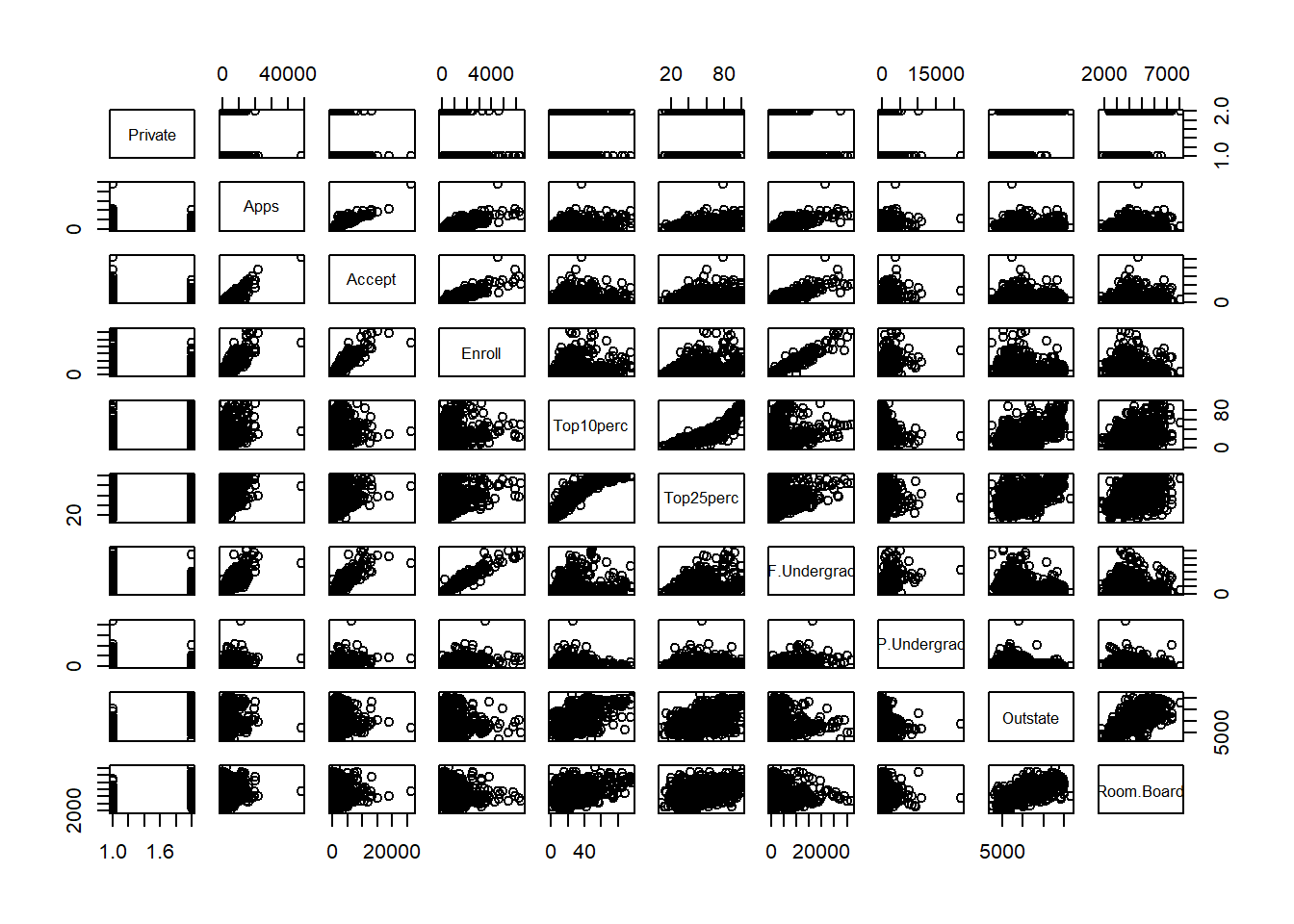From the matrix plot, we see that there is a clear positive trend between Top10perc and Top25perc, Enroll and F.Undergrad, Apps and Accept, and Accept and Enroll.

First we will look at the application and admission process for the colleges. Inspection of the admission related variables (Number of applications, number of acceptances, number of enrollments, Top 10%, Top 25% , number of Full Time Undergraduates and number of Part Time Undergraduates) shows that there is an outlier in number of applications. This point is Rutgers University at New Brunswick which received 48,094 applications, more than double the next highest University which had 21,804 applications. It is unknown whether this is an error in the data, or if there is a legitimate reason for the high value. For the purpose of this analysis, we will exclude the point for the admissions data analysis.

As we’d expect, there are generally positive correlations between number of applications, number of students accepted, and number of students enrolled. More students are applying to Colleges with higher acceptance rates.

Colleges with a higher percentage of students from the top 25 percent of the high school class had a relatively flat number of applications. There is not a clear relationship or any indication that applicants are applying more to elite schools over non-elite schools. Part time undergraduates has a potential outlier, University of Minnesota Twin Cities, with 21,836 Part-time undergraduates. While there are 36 colleges listed with more Part time undergrads than Full time, UMN Twin Cities has by far the largest number of undergraduates. Apart from this, there are no clear trends among the other variables.

Next we will look at the cost of the college. To focus on tuition, the out of state tuition is higher for private colleges than public, as we would expect.

``plot(College\$Private, College\$Outstate, xlab = "Private University", ylab = "Out-of-State Tuition Cost")``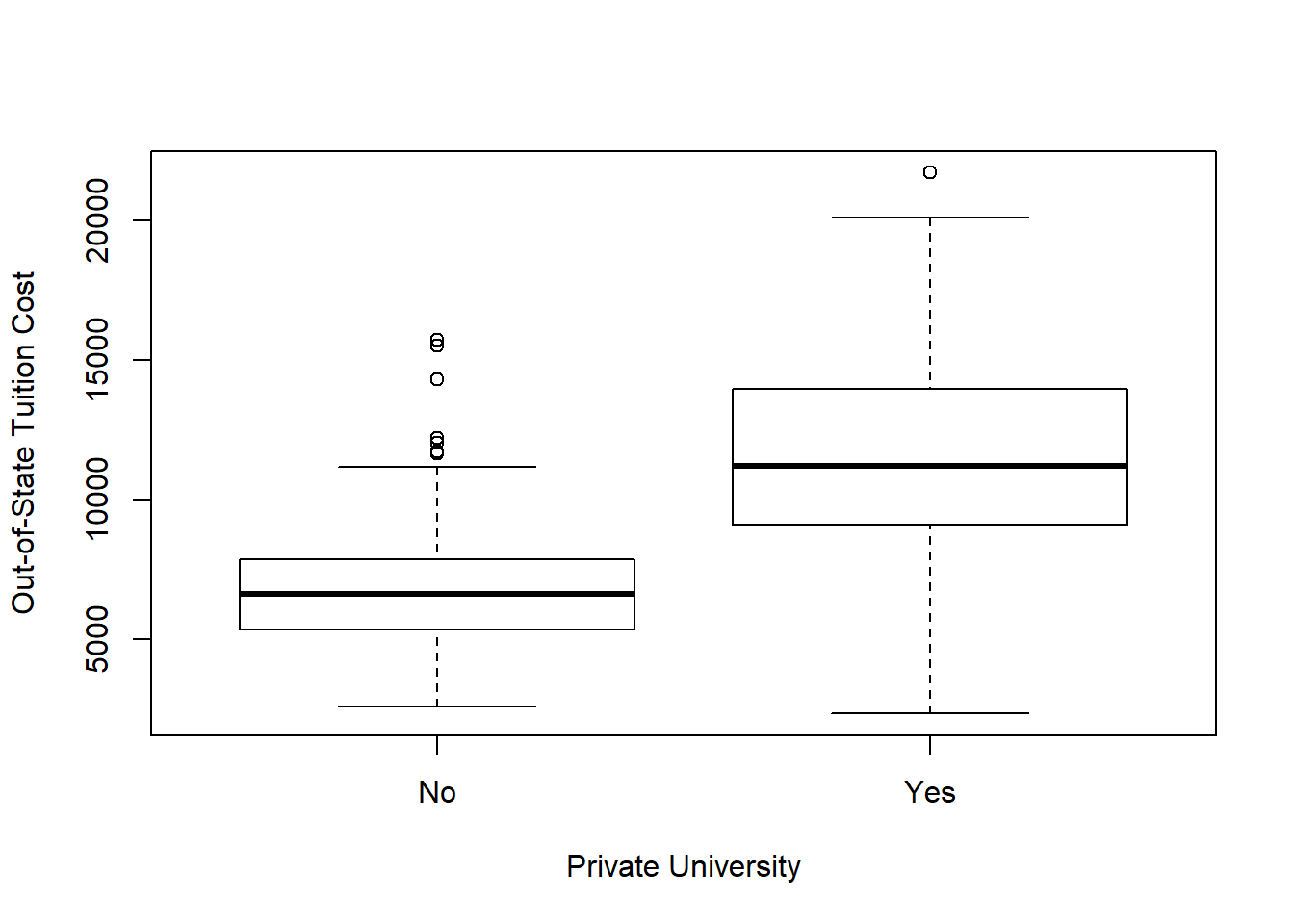We see that the tuition is on average more expensive at a Private university compared to a public university.

The mean public out of state tuition is \$6,813 compared to private college mean out of state tuition of \$11,801.

We will now create a new qualitative variables called ‘Elite’, by binning the Top10perc variable. We are going to divide universities into two groups based on whether or not the proportion of students coming from the top 10% of their high school classes exceeds 50%.

We Will initialize a column vector with the same number of observations as in the data.

``Elite <- rep("No", nrow(College))``

Now will conditionally select the elements of the vector ‘Elite’ to set to “Yes” based on whether or not Top10perc > 50 for each observation

``Elite[College\$Top10perc > 50] = 'Yes'``

Now time to turn Elite into a categorical variable with ‘Yes’ and ‘No’ as levels.

``Elite = as.factor(Elite)``

Append the ‘Elite’ variable to the College data set.

``College2 <- data.frame(College, Elite)``

Let’s visualize the out-of-state for Elite vs Non-Elite universities.

``plot(College2\$Elite, College2\$Outstate, xlab = "Elite", ylab = "Out-of-State Tuition Cost")``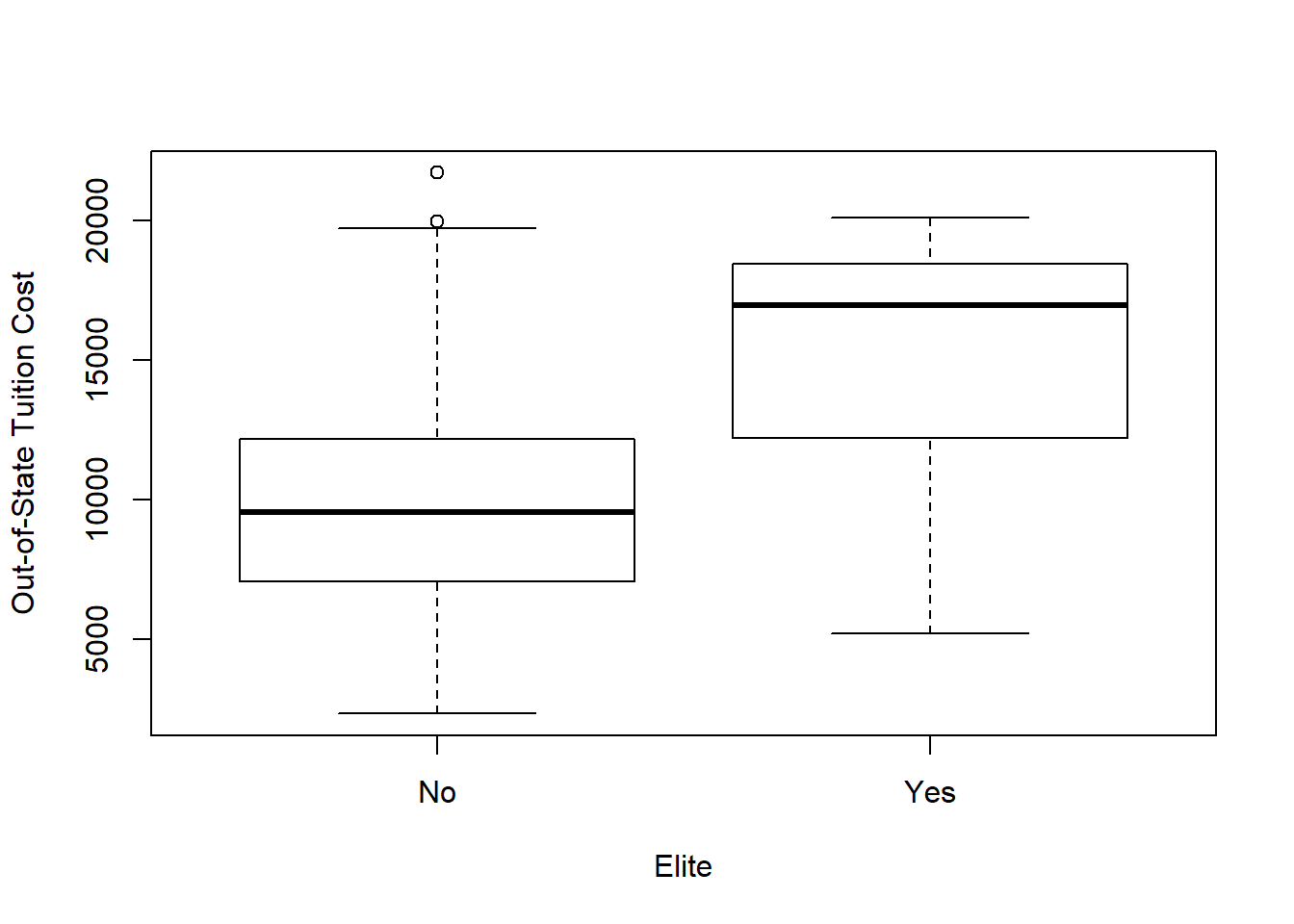On average the out-of-state tuition is higher at ‘Elite’ colleges vs ‘Non-Elite’ colleges. For Elite schools, the mean tuition is \$15,249 compared to \$9,904 for non-Elite Colleges. Overall, out of state tuition has a slight right skew. 50% of colleges’ out of state tuition is between \$7,305 and \$12,931, with the highest tuition being \$21,700, more than double the median tuition.

Let us get an overview of our newly created variable ‘Elite’.

``summary(Elite)``
``````##  No Yes
## 699  78``````

There seem to be only 78 Universities with more than 50% of students who were in the top 10% of their High School class.

``````par(mfrow=c(2,2))
hist(College[,9], main = "Out of State Tuition", xlab = "")
hist(College[,10], main = "Room & Board", xlab = "")
hist(College[,11], main = "Est. Book Costs", xlab = "")
hist(College[,12], main = "Est. Personal Spending", xlab = "")``````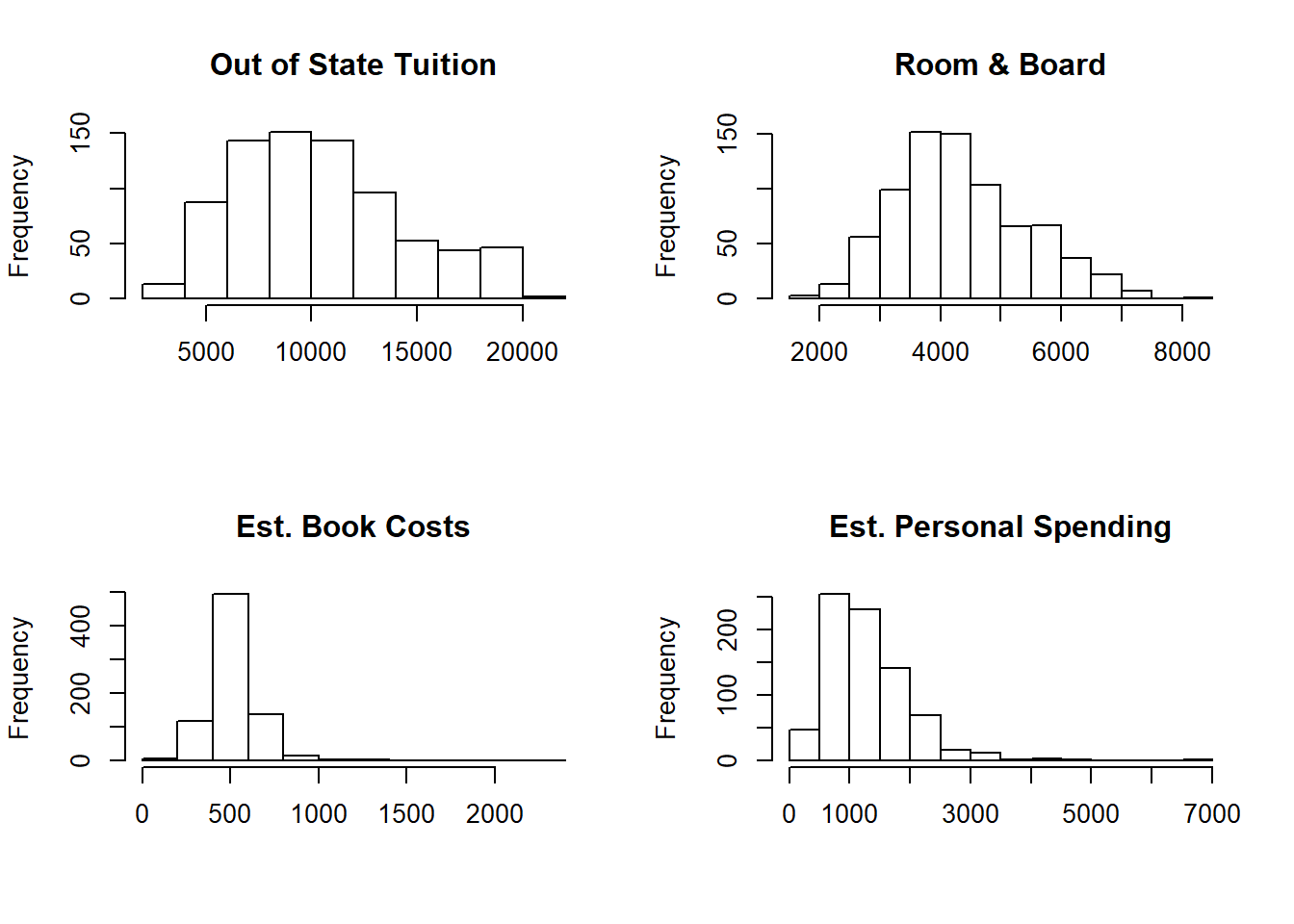All four main spend categories have a right skew, the most exaggerated being personal spending with a potential outlier in the highest bin. This is St. Louis University, a Private college with an estimated personal spending of \$6,800 compared with a median personal spending estimate of \$1,200.

To compare the cost of college to the student, we will define a variable for Total Payment as the sum of a student’s estimated costs: Out of State tuition + Room & Board + estimated cost of Books + estimated personal spending. We will use this variable in analysis to compare qualities of the education against the cost. The quality variables in the data set include the Student-Faculty ratio, percentage of Alumni donating to the college, college expenditure per student, and graduation rate. Distributions of these variables are shown below.

``````par(mfrow = c(2,2))
hist(College\$S.F.Ratio, main = "Student-Faculty Ratio", xlab = "")
hist(College\$perc.alumni, main = "Percentage of Alumni Donating", xlab = "")
hist(College\$Expend, main = "Expenditure per student", xlab = "")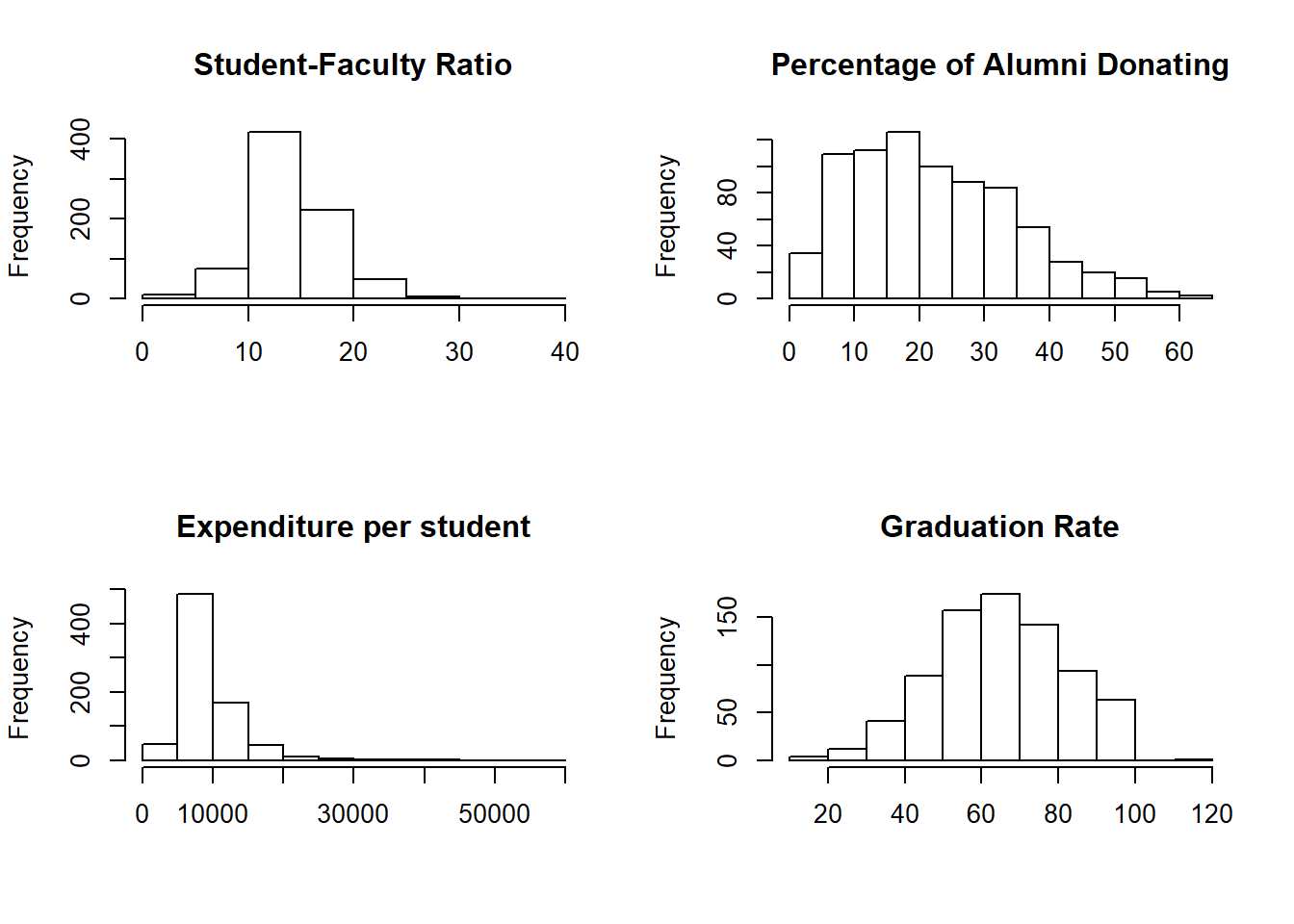The distribution of Graduation Rate shows one college, Cazenovia College, with a graduation rate of 120%. This is possibly a mistake; we will need to understand how the metric is calculated and how a rate of greater than 100% is possible.

We see a long tail on the expenditure per student. Most colleges spend between \$5,000-10,000 per student but is as high as \$56,000 per student at Johns Hopkins University. Compared to tuition, there is only a small increase in expenditure per student in the lower end ofthe scale for tuition, with a steep increase showing only at the highest tuition. Colleges with a high tuition will be generating more revenue per student to spend, but at the lower ranges, there appears to be little difference between the expenditure perr student. Further research should be done to understand what benefit a student is getting from a higher tuition in this low range. To take two colleges with similar expenditure as an example, a student at Auburn University-Main Campus pays a tuition of \$6,300, with a college expenditure of \$6,642. A student at Franklin Pierce College pays more than double the tuition at \$13,320 with a lower college expenditure of \$6,418. In depth research would be required to understand what the additional tuition represents in terms of educational quality.

``````totalpayment = College\$Outstate+College\$Books+College\$Room.Board+College\$Personal
College = cbind(College, totalpayment)``````
``````plot(College\$Grad.Rate~College\$totalpayment, ylab = "Graduation Rate", xlab = "Total Cost",
main = "Graduation Rate versus Cost")
abline(model)``````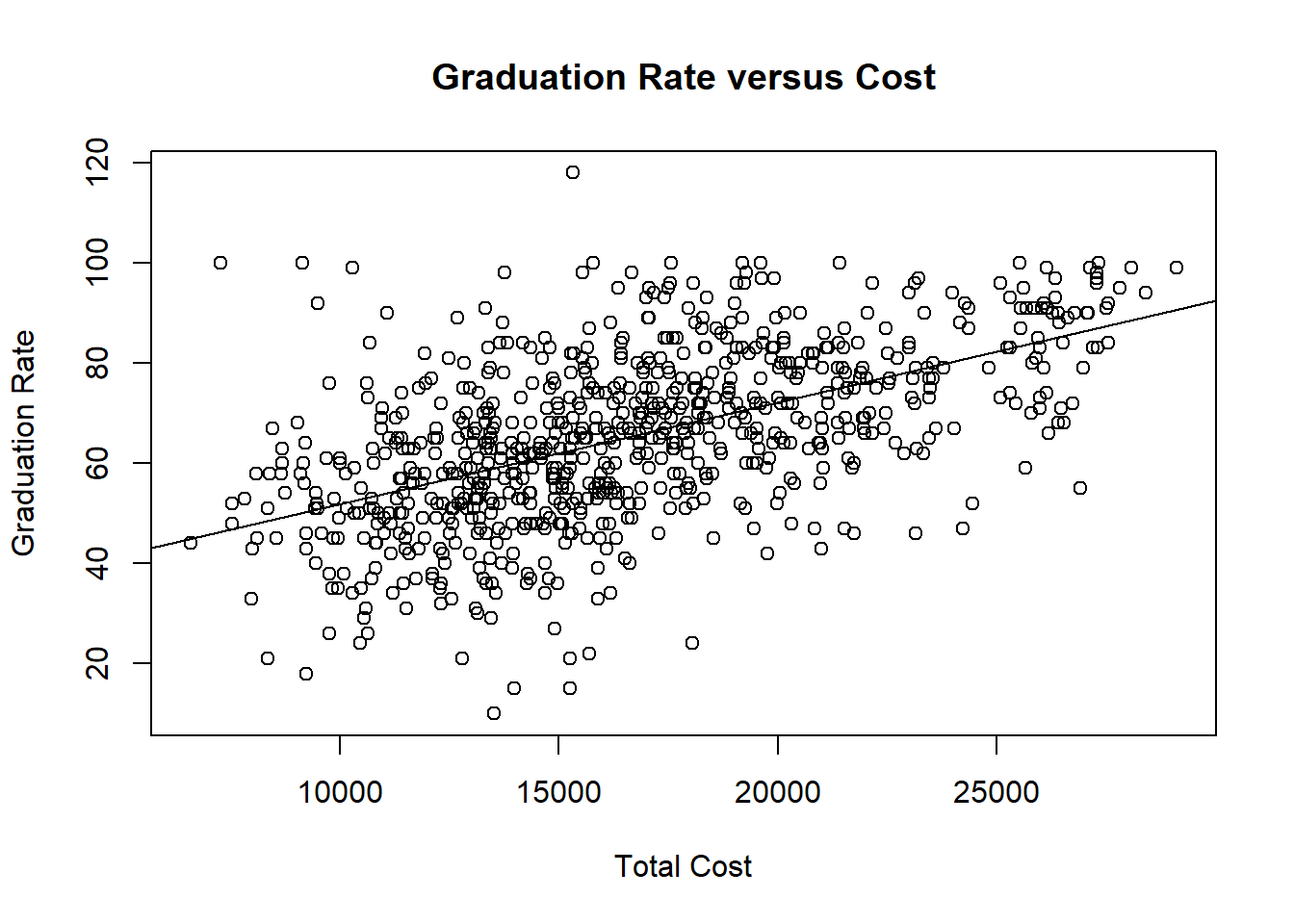A higher expenditure does not necessarily indicate a higher graduation rate, as seen below. There is an increase in graduation rate with cost, however we still see a band of colleges with a graduation rate above 80% even at the lower cost.

Let us see the histograms for a few insteresting variables:

``hist(College\$Apps,main = 'Histogram of Number of Applications', xlab = 'Applications')``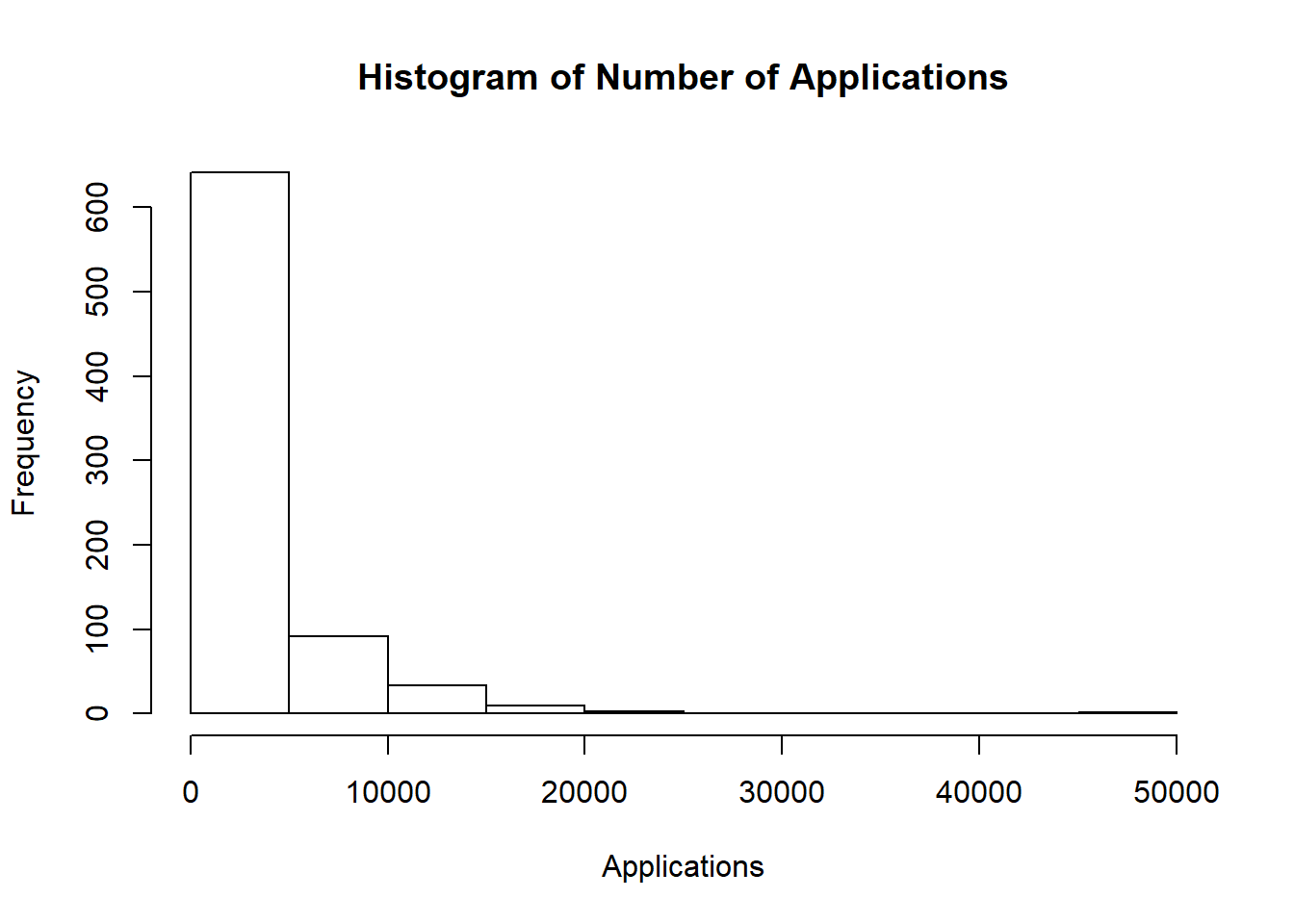First, let us check out a histogram of ‘Apps’ which is the number of applications received. It will give us a general idea of how many applications schools receive. From the Application Histogram, we see that the number of applications is right skewed. Also we can see that most colleges received around ~5000 applications then the amount of colleges who receive more than that drops significantly.

``hist(College\$Grad.Rate,main = 'Histogram of Graduation Rates', xlab = 'Graduation Rates')``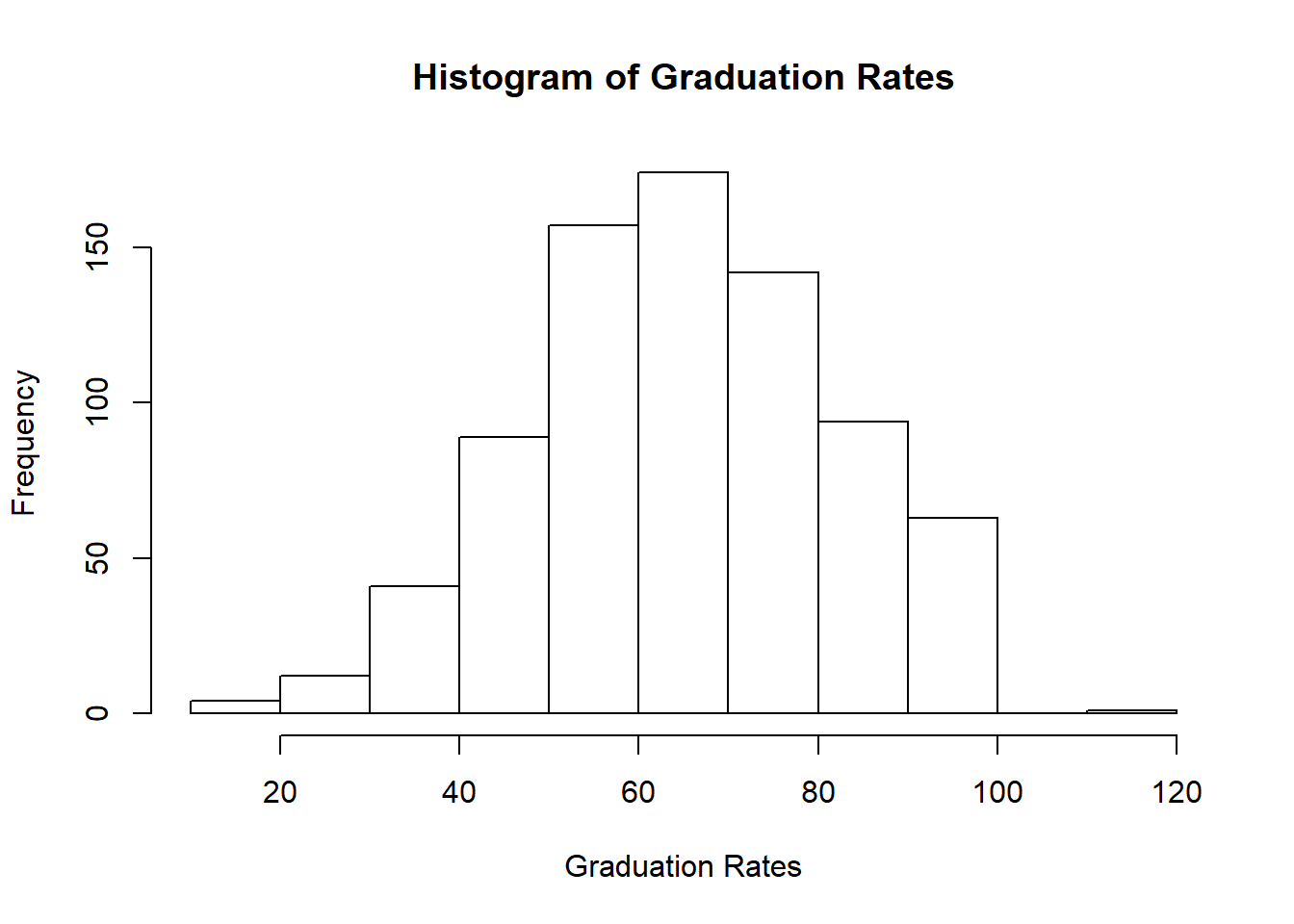From the histogram of Graduation Rates, we see what the graduation rates are for universities. This is interesting to see because we get a sense of what the general trend of graduation rates are across universities. From the plot, we see that there is an approximately normal distribution with a mean of around 60-70.

``hist(College\$Books,main = 'Histogram of Book Costs', xlab = 'Cost of Books')``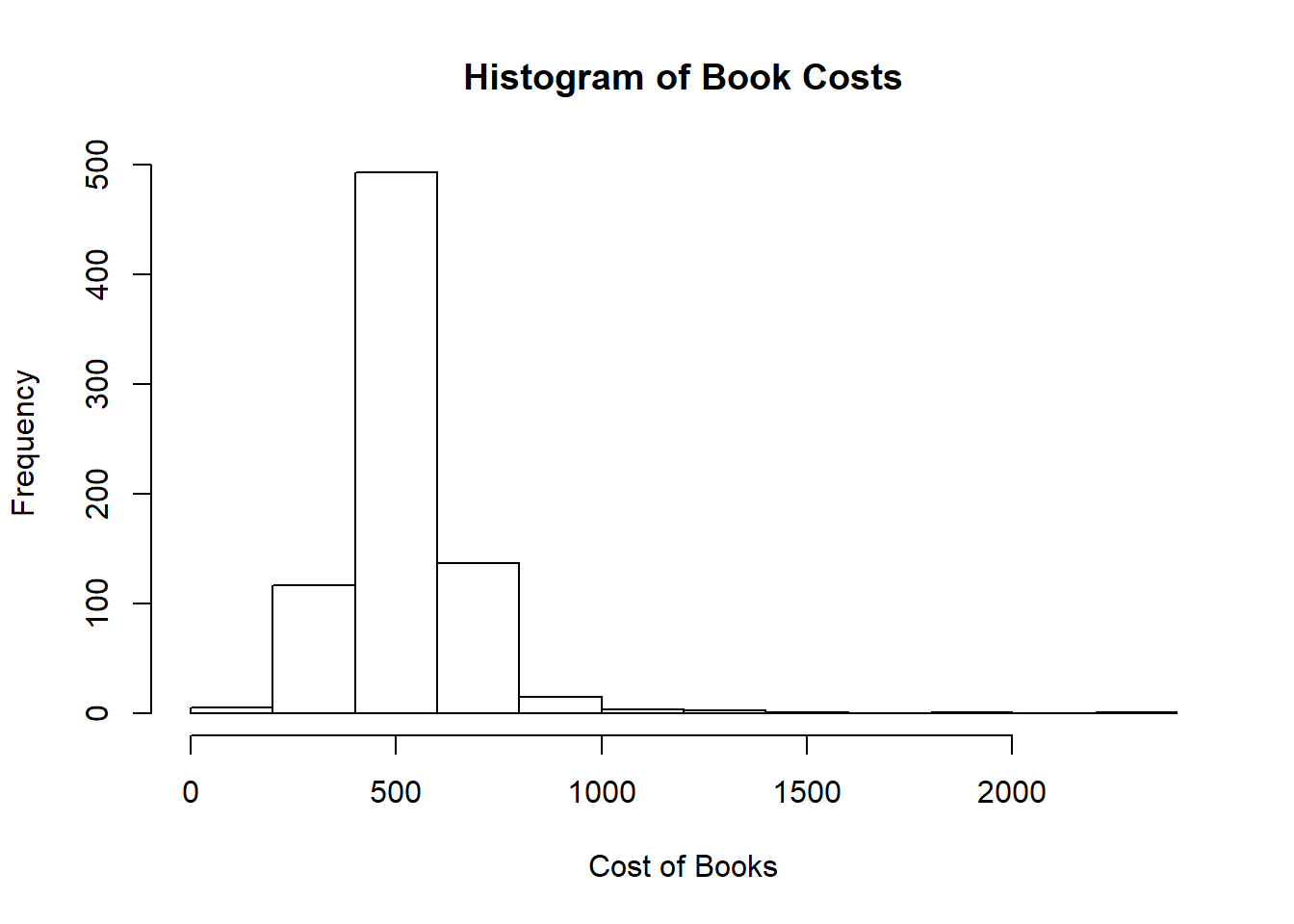Now let us investigate the ‘Book’ variable, which is the estimated cost of books. From the histogram, we see that there appears to not be a lot of variation in the amount of cost of books, as most of the data points are concentrated around 500.

``hist(College\$PhD,main = 'Histogram of Percent of Faculty with PhDs', xlab = 'Faculty with PhD')``